Test: Multiplication And Division - 2

# Test: Multiplication And Division - 2

Test Description

## 10 Questions MCQ Test Mathematics for Class 4: NCERT | Test: Multiplication And Division - 2

Test: Multiplication And Division - 2 for Class 4 2023 is part of Mathematics for Class 4: NCERT preparation. The Test: Multiplication And Division - 2 questions and answers have been prepared according to the Class 4 exam syllabus.The Test: Multiplication And Division - 2 MCQs are made for Class 4 2023 Exam. Find important definitions, questions, notes, meanings, examples, exercises, MCQs and online tests for Test: Multiplication And Division - 2 below.
Solutions of Test: Multiplication And Division - 2 questions in English are available as part of our Mathematics for Class 4: NCERT for Class 4 & Test: Multiplication And Division - 2 solutions in Hindi for Mathematics for Class 4: NCERT course. Download more important topics, notes, lectures and mock test series for Class 4 Exam by signing up for free. Attempt Test: Multiplication And Division - 2 | 10 questions in 20 minutes | Mock test for Class 4 preparation | Free important questions MCQ to study Mathematics for Class 4: NCERT for Class 4 Exam | Download free PDF with solutions
 1 Crore+ students have signed up on EduRev. Have you?
Test: Multiplication And Division - 2 - Question 1

### If the cost of 1 packet of TIGER biscuits is × 5, how many packets can I get for × 30 with the FREE OFFER shown here?Detailed Solution for Test: Multiplication And Division - 2 - Question 1

30 ÷ 5 = 6 packets with the offer. One can get 6 + 3 = 9 biscuit packets free.

Test: Multiplication And Division - 2 - Question 2

### If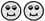Stands of 30, for which number will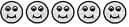stand?

Detailed Solution for Test: Multiplication And Division - 2 - Question 2

If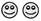stands for 30, each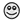stands for 15. So,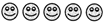stands for 5 ×15 = 75

Test: Multiplication And Division - 2 - Question 3

### Study the given equations.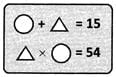If these number sentences are true, which of the following is correct?

Test: Multiplication And Division - 2 - Question 4

Navya wants to read a book which has a total of 340 pages. Of these, 16 pages have only drawings and another 6 pages contain the author's note, contents etc. If each remaining page has around 30 lines of print, what is the total number of lines that Navya has to read?

Test: Multiplication And Division - 2 - Question 5

Twice a certain number is 42.What is six times that number?

Test: Multiplication And Division - 2 - Question 6

If the product of all the three numbers in any row, column or diagonal is the same, find the missing number in the given square.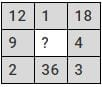Test: Multiplication And Division - 2 - Question 7

A What is the value (137 × 137) − (136 × 136)?

Detailed Solution for Test: Multiplication And Division - 2 - Question 7

Observe the following. We have, (2 × 2) − (1 × 1) = 3 = 2 + 1 (3 × 3) − (2 × 2)= 5 = 3 + 2 (5 × 5) − (4 × 4) = 9 = 5 + 4 (12 × 12) −(11 × 11) = 23 = 12 + 11 Similarly, (137 × 137) −(136 × 136) = 137 + 136 = 273

Test: Multiplication And Division - 2 - Question 8

In the given multiplication, the product 11418 is incorrect due to a mistake in one of the digits.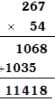Find the incorrect digit.

Test: Multiplication And Division - 2 - Question 9

If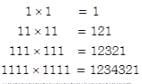find the product of 11111 × 11111.

Detailed Solution for Test: Multiplication And Division - 2 - Question 9

11111 × 11111 = 123454321

Test: Multiplication And Division - 2 - Question 10

Study the following diagram and find the missing term.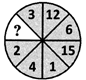Detailed Solution for Test: Multiplication And Division - 2 - Question 10

1 × 3 = 3, 4 × 3 = 12, 2 × 3 = 6 Similarly, 5 × 3 = 15.

## Mathematics for Class 4: NCERT

24 videos|44 docs|32 tests
 Use Code STAYHOME200 and get INR 200 additional OFF Use Coupon Code
Information about Test: Multiplication And Division - 2 Page
In this test you can find the Exam questions for Test: Multiplication And Division - 2 solved & explained in the simplest way possible. Besides giving Questions and answers for Test: Multiplication And Division - 2, EduRev gives you an ample number of Online tests for practice

## Mathematics for Class 4: NCERT

24 videos|44 docs|32 tests